## Inequalities

An inequality is a statement in which the relationships are not equal. Instead of using an equal sign (=) as in an equation, these symbols are used: > (is greater than) and < (is less than) or ≥ (is greater than or equal to) and ≤ (is less than or equal to).

#### Axioms and properties of inequalities

For all real numbers a, b, and c, the following are some basic rules for using the inequality signs.

• Trichotomy axiom: a > b, a = b, or a < b.

These are the only possible relationships between two numbers. Either the first number is greater than the second, the numbers are equal, or the first number is less than the second.

• Transitive axiom: If a > b, and b > c, then a > c.

Therefore, if 3 > 2 and 2 > 1, then 3 > 1.

If a < b and b < c, then a < c.

Therefore, if 4 < 5 and 5 < 6, then 4 < 6.

• If a > b, then a + c > b + c.

• If a > b, then ac > bc.

• If a < b, then a + c < b + c.

• If a < b, then ac < bc.

Adding or subtracting the same amount from each side of an inequality keeps the direction of the inequality the same.

Example: If 3 > 2, then 3 + 1 > 2 + 1 (4 > 3)

If 12 < 15, then 12 – 4 < 15 – 4 (8 < 11)

• Positive multiplication and division property:

• If a > b, and c > 0, then ac > bc.

• If a < b, and c > 0, then ac < bc.

• If a > b, and c > 0, then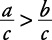.

• If a < b, and c > 0, then.

Multiplying or dividing each side of an inequality by a positive number keeps the direction of the inequality the same.

Example: If 5 > 2, then 5(3) > 2(3), therefore, 15 > 6.

If 3 < 12, then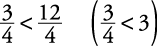.

• Negative multiplication and division property:

• If a > b, and c < 0, then ac < bc.

• If a < b, and c < 0, then ac > bc.

• If a > b, and c < 0, then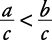.

• If a < b, and c < 0, then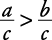.

Multiplying or dividing each side of an inequality by a negative number reverses the direction of the inequality.

Example: If 5 > 2, then 5(– 3) < 2(– 3); therefore, – 15 < – 6.

If 3 < 12, then.

#### Solving inequalities

When working with inequalities, treat them exactly like equations (except, if you multiply or divide each side of the inequality by a negative number, you must reverse the direction of the inequality).

##### Example 1

Solve for x: 2 x + 4 > 6.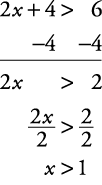Answers are sometimes written in set builder notation { x: x > 1}, which is read “the set of all x such that x is greater than 1.”

##### Example 2

Solve for x: –7 x > 14.

Divide by –7 and reverse the direction of the inequality.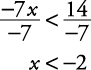##### Example 3

Solve for x: 3 x + 2 ≥ 5 x – 10.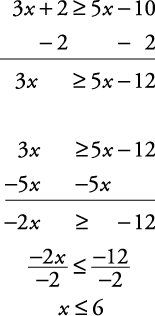Notice that opposite operations are used. Divide each side of the inequality by –2 and reverse the direction of the inequality.

In set builder notation, { x: x ≤ 6}.